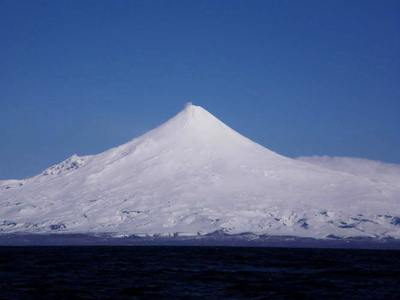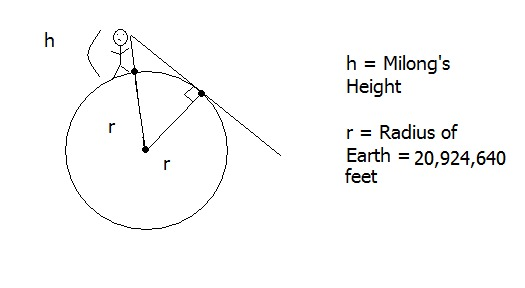# How far is the horizon?

Alignments to Content Standards: G-MG.A.1 G-SRT.B.5 G-MG.A

Milong and her friends are at the beach looking out onto the ocean on a clear day and they wonder how far away the horizon is.

1. About how far can Milong see out on the ocean?
2. If Milong climbs up onto a lifeguard tower, how far is the horizon in Milong's view?
3. Mount Shishaldin lies on a narrow peninsula in Alaska and is pictured here:If Milong were to stand atop Mount Shishaldin and look out over the ocean, how far would the horizon be?

4. Based on the answers to the questions above, is the distance of Milong's visual horizon proportional to her elevation above the surface of the earth? Explain.

## IM Commentary

The purpose of this modeling task is to have students use mathematics to answer a question in a real-world context using mathematical tools that should be very familiar to them. The task gets at particular aspects of the modeling process, namely, it requires them to make reasonable assumptions and find information that is not provided in the task statement. Students will need to make an assumption about Milong's height and the height of the lifeguard tower and assume, particularly for part (c), that it is clear enough to actually see all the way to the horizon. They will also need to look up the height of Mt. Shishaldin, although the teacher could give them this information if the students do not have access to the internet. In any case, the teacher should have all necessary information ready in case students are struggling to get started on the problem.

This task assumes that students are well-versed in the use of the Pythagorean Theorem and know what a tangent line to a circle is, although the task does not explicitly tell them that these are the proper mathematical tools. In addition, in order to apply the Pythagorean Theorem, students must know that the tangent line to a circle meets the radius at the point of tangency in a right angle. If students don't understand why the tangent line is the best way to represent the line-of-sight to the horizon, they might benefit from exploring this Desmos sketch:

Ideas and mathematical work closely related to this problem are presented in

and

The inverse problem, namely how high do you have to be in order to see a certain distance, is also important and is developed here:

The mathematics is very similar but the given information and the unknown change in the two scenarios.

This task supports two of the standards of mathematical practice:

• MP1, Make Sense of Problems and Persevere in Solving Them: students need to make reasonable assumptions about Milong's height and the height of the lifeguard tower and must look up the height of Mount Shishaldin. In addition, no indication is given how to estimate the length of Milong's line of sight which will require creativity and skill.
• MP4, Model with Mathematics: in order to estimate the length of Milong's line of sight, students will need to approximate the earth with a sphere and then use their geometric knowledge in order reduce the computation to an application of the Pythagorean Theorem.

This task was designed for an NSF supported summer program for teachers and undergraduate students held at the University of New Mexico from July 29 through August 2, 2013 (http://www.math.unm.edu/mctp/). A group of participants in the program wrote the solution including the pictures.

## Solution

1. Because the earth is round, our line of sight terminates at some point on the horizon. That line of sight is the tangent line to the curve of the earth. This tangent line touches the circle at a single point and is perpendicular to the radius at that point.

Because we don't know how tall Milong is, we'll need make a reasonable assumption about her height. Let's assume her eyes are 5 feet above the surface of the ocean. According to Wikipedia, the radius of the earth is 3,959 miles or 3959 $\times$ 5280 = 20,924,640 feet.

Next, we can draw an idealized picture of Milong standing on the surface of the earth with her line of sight terminating at some point on the curve of the earth (which we assume is a sphere). Milong's height is greatly exaggerated in the picture in order to see clearly all of the quantities we need to solve the problem.Notice that the radius is perpendicular to the tangent line giving us a right triangle as indicated in the picture below:Now, we may use the Pythagorean Theorem to find the length of Milong’s line of sight (labeled $B$ in the picture).

\begin{align} A^2 + B^2 =& C^2 \\ B^2 =& C^2 - A^2 \\ B^2 =& (20,924,640+5)^2 - (20,924,640)^2 \\ B =& \sqrt{(20,924,640+5)^2 - (20,924,640)^2} \end{align}
So $B\approx 14,465$ feet and $$14,465 \text{ feet} \times (1 \text{ mile}/5280 \text{ feet}) \approx 2.7 \text{ miles.}$$ Thus, Milong can see about 2.7 miles out on the ocean if her eyes are 5 feet above the surface of the ocean.
2. If Milong climbs up onto a lifeguard tower, this solution follows the above steps with the same radius. However, $h$ is now the height of Milong plus the height of the lifeguard tower (assuming she is standing on the platform of the lifeguard tower). Let’s assume the platform for the lifeguard tower is 10 feet high. Then we can calculate the length of Milong's line of sight as above:

\begin{align} A^2 + B^2 =& C^2 \\ B^2 =& C^2 - A^2 \\ B^2 =& (20,924,640+5+10)^2 - (20,924,640)^2 \\ B =& \sqrt{(20,924,640+5+10)^2 - (20,924,640)^2} \end{align}

So $B\approx 25,054.7$ feet and $$25,054.7 \text{ feet} \times (1 \text{ mile}/5280 \text{ feet}) \approx 4.7 \text{ miles.}$$ So Milong can see about 4.7 miles out on the ocean when standing on a 10-foot lifeguard tower.

3. When Milong is standing at the top of Mount Shishaldin (9373 feet), the steps are the same as parts (a) and (b) with a change in height $h$, which is now the height of Milong plus the height of Mt. Shishaldin. A Google search reveals the height of Mt. Shishaldin is 9373 feet. We can now repeat the calculation above to find the length of Milong's line of sight:

\begin{align} A^2 + B^2 =& C^2 \\ B^2 =& C^2 - A^2 \\ B^2 =& (20,924,640+5+9373)^2 - (20,924,640)^2 \\ B =& \sqrt{(20,924,640+5+9373)^2 - (20,924,640)^2} \end{align}
So $B\approx 626,538$ feet and $$626,538 \text{ feet} \times (1 \text{ mile}/5280 \text{ feet}) \approx 118.7 \text{ miles.}$$ So Milong could see about 120 miles out on the ocean if she were standing on the top of Mt. Shishaldin.

4. As Milong’s height increases, the distance that she can see increases as well. However, looking at the following table:
Milong's height $h$ Distance to the horizon $B$ $h\div B$
5 2.7 1.85
15 4.7 3.19
9378 120 78.15
We see that Milong's height above the surface of the earth and the distance that she can see to the horizon are not proportional.